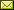## A Divergence Formula for Randomness and Dimension (Short Version)

 Jack H. Lutz

 If S is an infinite sequence over a finite alphabet Σ and β is a probability measure on Σ, then the \it dimension of S with respect to β, written \dim^β(S), is a constructive version of Billingsley dimension that coincides with the (constructive Hausdorff) dimension \dim(S) when β is the uniform probability measure. This paper shows that \dim^β(S) and its dual \Dim^β(S), the \it strong dimension of S with respect to β, can be used in conjunction with randomness to measure the similarity of two probability measures α and β on Σ. Specifically, we prove that the \it divergence formula $\dim^β(R) = \Dim^β(R) =\frac\CH(α)\CH(α) + \D(α || β)$ holds whenever α and β are computable, positive probability measures on Σ and R \in Σ^∞ is random with respect to α. In this formula, \CH(α) is the Shannon entropy of α, and \D(α||β) is the Kullback-Leibler divergence between α and β.

In Turlough Neary, Damien Woods, Tony Seda and Niall Murphy: Proceedings International Workshop on The Complexity of Simple Programs (CSP 2008), Cork, Ireland, 6-7th December 2008, Electronic Proceedings in Theoretical Computer Science 1, pp. 149–152.
Published: 25th June 2009.

 ArXived at: https://dx.doi.org/10.4204/EPTCS.1.14 bibtex PDF

 Comments and questions to:eptcs@eptcs.org For website issues:webmaster@eptcs.org